Final Race Results ~ 1998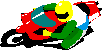The 1st section describes all abbreviations used on this page. The 2nd section lists my placings for each race competed in. The 3rd section lists my overall WSMC points standings after each race weekend throughout the year in each class..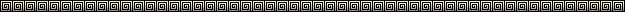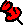1998 WSMC Championship Standings:  (Final)

 WSMC OVERALL: 15th 500 Singles: 3rd Formula Singles: 4th Lightweight Vintage: 3rd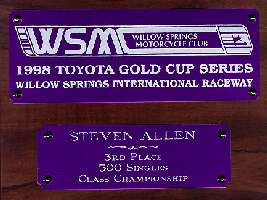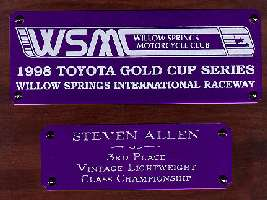Race classes will be abbreviated in the following manner:

FS = Formula Singles
500 = 500 Singles
V = Lightweight Vintage Dinosaurs
SS = 750 Super Stock
MP = 750 Modified Production
SB = 750 Super Bike
F1 = Formula 1
SM = Super Mono 1
SM2 = Super Mono 2
O/A = WSMC Over All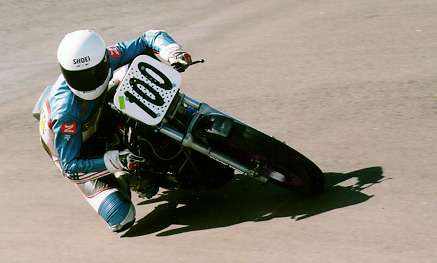The following are my placings for each & all races entered:

WSMC rnd 1:  500=4th, FS=7th, V=5th, F1=32nd
WSMC rnd 2: 500=3rd, FS=4th, V=4th, F1=21st
WSMC rnd 3:  500=2nd, FS=4th, V=6th
WSMC rnd 4:  500=6th, FS=5th, V=3rd
(crash in 500, finished last lap only for points)
AHRMA (Sears Point):
SAT: SM2=2nd, SM=5th. SUN: SM2=2nd, SM=4th
AHRMA (Moto Classica):
SAT: SM2=3rd, SM=7th. SUN: SM2=3rd, SM=7th
WSMC rnd 5: DNS ~
major mechanical during practice & couldn't make the starts
WSMC rnd 6:  500=3rd, FS=5th, V=5th
WSMC rnd 7:  500=2nd, FS=4th, V=3rd
WSMC rnd 8:  500=2nd, FS=4th, V=3rd
WSMC rnd 9:  500=2nd, FS=3rd, V=2nd
WSMC rnd 10:  500=1st, FS=4th, V=4th (first win for 1998)
WSMC rnd 11:   motor seized in first race - argh!
WSMC rnd 12:  500=3rd, FS=5th, V=4th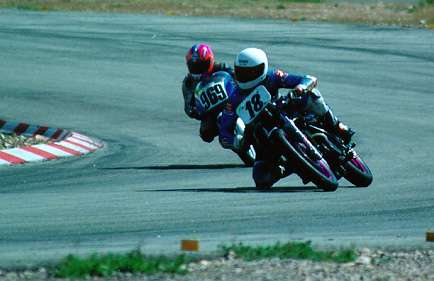The following are my WSMC OVERALL & class point standings after each event:

WSMC rnd 1:  500=4th, FS=7th, V=5th, F1=32nd, O/A=37th
WSMC rnd 2: 500=3rd (tied), FS=5th, V=4th, F1=15th (tied), O/A=30th (tied)
WSMC rnd 3: 500=2nd, FS=4th, V=5th, F1=18th (tied), O/A=20th (tied)
WSMC rnd 4: 500=4th, FS=4th, V=3rd (tied), F1=25th, O/A=15th
(Crash in 500 Singles)
WSMC rnd 5: 500=5th, FS=5th, V=4th, F1=33rd (tied), O/A=21st
(DNS all races)
WSMC rnd 6: 500=4th, FS=5th, V=4th, F1=35th (tied), O/A=22nd (tied)
WSMC rnd 7: 500=3rd, FS=5th, V=4th, F1=39th, O/A=16th
(double points round)
WSMC rnd 8: 500=3rd, FS=5th, V=4th, F1=41st, O/A=14th
WSMC rnd 9: 500=3rd, FS=5th, V=3rd, F1=45th, O/A=14th
(tied.double pts rnd)
WSMC rnd 10: 500=3rd, FS=4th, V=3rd, F1=?, O/A=13th
WSMC rnd 11: 500=3rd, FS=4th, V=3rd, F1=?, O/A=15th
WSMC rnd 12: 500=3rd, FS=4th, V=3rd, F1=?, O/A=15th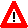All images, pictures & information contained within this website
property of &